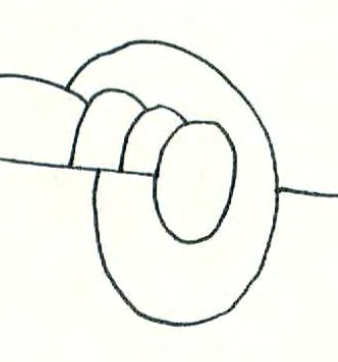# Map ColoringHenri Picciotto

These activities are based on lessons I used to teach decades ago in grades 4-5. They are presented here in a form suitable for high school or perhaps middle school.

Alternate version

Read no further if you want to work through the worksheets yourself!

## Teacher Notes

When I shared these activities with teachers at a conference, I was told they would work well in a high school discrete math class, as a way to introduce a graph theory unit.

The first activity in the packet introduces the idea of map coloring, and the second leads to the Two-Color Theorem. (Note that the word "line" is used informally, to mean any curve joining one vertex to another. The formal term is "edge".)

The key insight is that the only way to get to a two-color map by drawing additional edges is by doing it so that each vertex is surrounded by an even number of regions. One possible statement of the theorem is:

A map is a two-color map if all its vertices are even.

You may prefer to use different terminology, either for consistency with a textbook, or to incorporate vocabulary suggested by your students. In any case, it is essential to define terms so that the theorem makes sense.

A clarifying discussion could include:
- What about vertices on the boundary of the map?
- Does the theorem apply to maps on the whole plane? (as opposed to the finite maps given in the worksheets)
- What can be said about a map created by (infinite, straight) lines?
- What can be said about a map created by drawing only (possibly intersecting) closed curves (e.g. circles, ellipses, polygons, figure eights, etc.)?
- What about combinations of the above two?

### Animated Riddle

I scripted a version of these activities for a TED-Ed animated riddle. I recommend only showing this to your students after doing the activities I shared above, because the riddle only includes two maps, which may not be sufficient for students to sort out what's going on. On the other hand, after they have done the activities, they probably would enjoy the animation, and appreciate the clarity of the explanations that follow the pause. (However, note that there is an ad at the end, starting at 4:50.)

You can get the riddle maps as Google Draw documents.

### Extension

These activities can precede a discussion of the Konigsberg Bridge problem. (If you are not familiar with it, a Web search should yield many links.)